# Non Verbal Reasoning - Analogy

### Exercise :: Analogy - Section 2

Each of the following questions consists of two sets of figures. Figures A, B, C and D constitute the Problem Set while figures 1, 2, 3, 4 and 5 constitute the Answer Set. There is a definite relationship between figures A and B. Establish a similar relationship between figures C and D by selecting a suitable figure from the Answer Set that would replace the question mark (?) in fig. (D).

1.

Select a suitable figure from the Answer Figures that would replace the question mark (?).

Problem Figures:                            Answer Figures: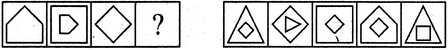(A)     (B)      (C)     (D)                  (1)      (2)      (3)      (4)      (5)

 A. 1 B. 2 C. 3 D. 4 E. 5

Explanation:

The figure rotates 90oCW; gets reduced in size and also gets enclosed in a figure with one less number of sides.

2.

Select a suitable figure from the Answer Figures that would replace the question mark (?).

Problem Figures:                            Answer Figures: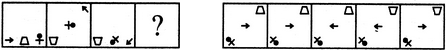(A)     (B)      (C)     (D)                  (1)      (2)      (3)      (4)      (5)

 A. 1 B. 2 C. 3 D. 4 E. 5

Explanation:

The symbols move in the sequence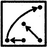. Also, the arrow rotates 135oACW; the trapezium gets vertically inverted and the pin-shaped symbol rotates 90oCW.

3.

Select a suitable figure from the Answer Figures that would replace the question mark (?).

Problem Figures:                            Answer Figures: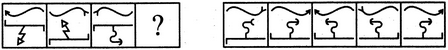(A)     (B)      (C)     (D)                  (1)      (2)      (3)      (4)      (5)

 A. 1 B. 2 C. 3 D. 4 E. 5

Explanation:

The upper element rotates through 180o and its head gets inverted. The lower element gets vertically inverted.

4.

Select a suitable figure from the Answer Figures that would replace the question mark (?).

Problem Figures:                            Answer Figures:(A)     (B)      (C)     (D)                  (1)      (2)      (3)      (4)      (5)

 A. 1 B. 2 C. 3 D. 4 E. 5

Explanation:

The figure gets laterally inverted.

5.

Select a suitable figure from the Answer Figures that would replace the question mark (?).

Problem Figures:                            Answer Figures: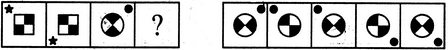(A)     (B)      (C)     (D)                  (1)      (2)      (3)      (4)      (5)

 A. 1 B. 2 C. 3 D. 4 E. 5